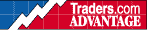HOT TOPICS LIST

INDICATORS LIST

LIST OF TOPICS

# Demand Index

04/02/00 02:12:33 PM
by Jason K. Hutson

Demand Index

Security:   N/A
Position:   N/A

 The study calculates the Buying Pressure (BP) and Selling Pressure (SP) in the following manner for theNo Limit version. If the prices rise: BP = V or VolumeSP = V/P where P is the percent change in priceIf the price declines: BP = V/P where P is the percent change in price If the price declines: SP = VBecause P is a decimal (i.e., less than 1), P is modified to make it greater than one by multiplying it bythe constant K. For the No Limit version K=(3*C)/Va where C is the closing price and Va is the Volatility average which is the ten-day average of a two-dayprice range (highest high minus lowest low).Also if SP> BP then DI = -BP/SP

Jason K. Hutson

Traders.com Staff Writer. Enjoys trendlines, support and resistance, moving averages, RSI, MACD, ADX, Bollinger bands, parabolic SAR, chart formations, and volume analysis.

 Comments or Questions? Article Usefulness 5 (most useful) 4 3 2 1 (least useful)